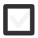# ALGEBRA. What is x in (x+2)/4-(x-2)/6=5? What is 0.25 + 1/2? What is 4/3 + 5/2?422 views
In 7-9
edited

## 2 lessons

Functions

Have you ever come across the problem of understanding how warm or cold it is when the temperature is given in Fahrenheit or vice versa, if you live in the US, in Celsius? Wouldn’t it be convenient if you could have a machine that would do the conversion for you? This is what functions do: You input something, and it is given as output as something else according to a rule.

Let’s learn the theory:

Excercises:

1. A function subtracts 9 from a number. What is the output of the function when input is 15? 45? 9? 5?

2. Calculate ƒ(x) = 5x + 1 when x = 7
x = 10
x = -1
x = -7

3. Calculate ƒ(x) = -3x + 10 when
x = 2
x = 12
x = 0
x = -6

3. For ƒ(x) = x – 6, which value of x gives the output of
5
0
-4
-12